# The 2016 Atomic Mass Evaluation (AME2016)

Atomic mass list for analysis which contains the elements, mass excess, binding energy, beta-decay energy, atomic mass and more

## Details

As a fundamental property of nuclei, atomic masses are widely used in many domains of science and engineering. A reliable atomic mass table derived from the experimental data, where the atomic masses and the relevant experimental information can be found conveniently, is in high demand by the research community. To meet the demands, the Atomic Mass Evaluation (Ame) was created in 1950’s and now serves the research community by providing the most reliable and comprehensive information related to the atomic masses. (G. Audi, Int. J. Mass Spectr.251: 85 (2006) http://dx.doi.org/10.1016/j.ijms.2006.01.04). This data represents the newest published one in 2016. It is called AME2016.

## Examples

### Basic Examples

 In:=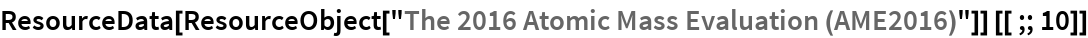Out=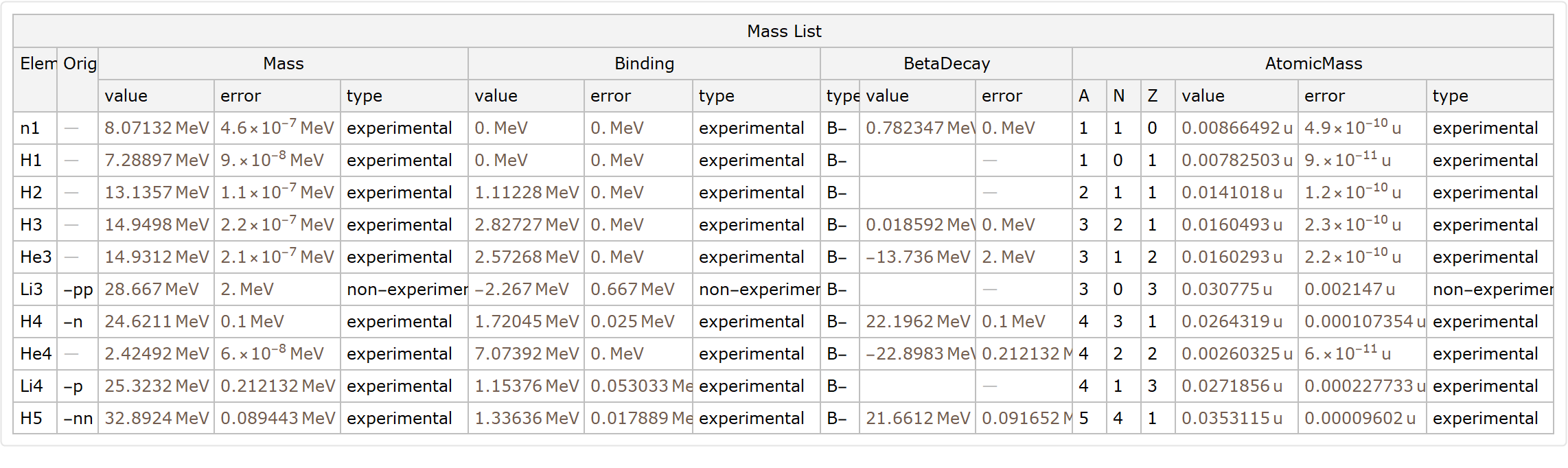### Visualizations

Retrieving one element:

 In:=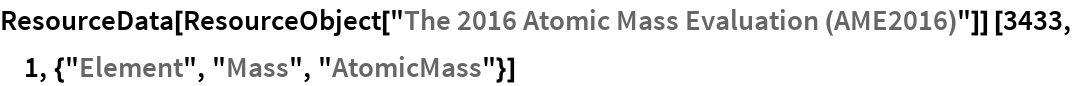Out=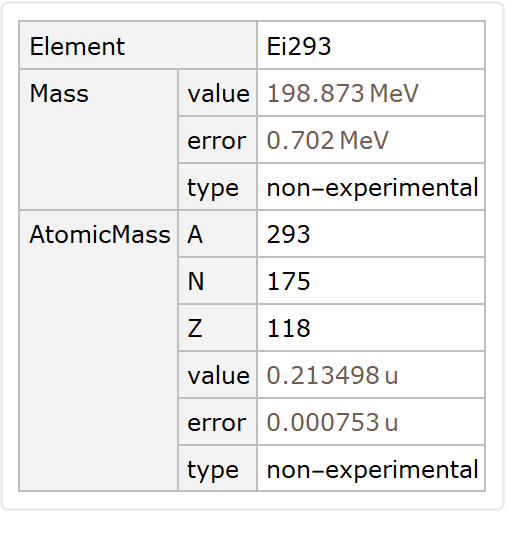Let' s compare this data with the one available at Wolfram Language. First we select some information from the resource data:

 In:=Out=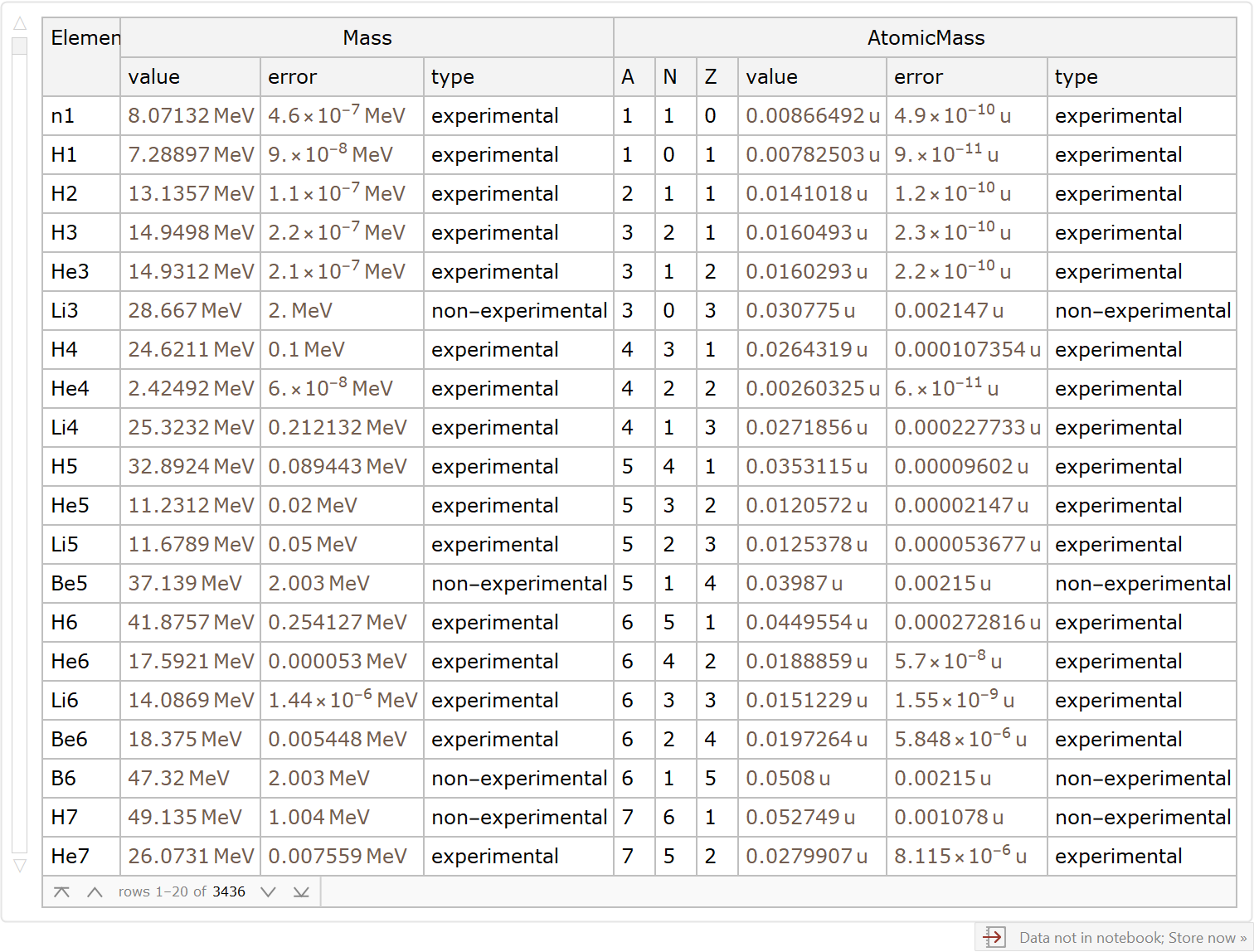Now, we retrieve the data that are listed as entities in Wolfram Language (we remove the neutron from the data):

 In:=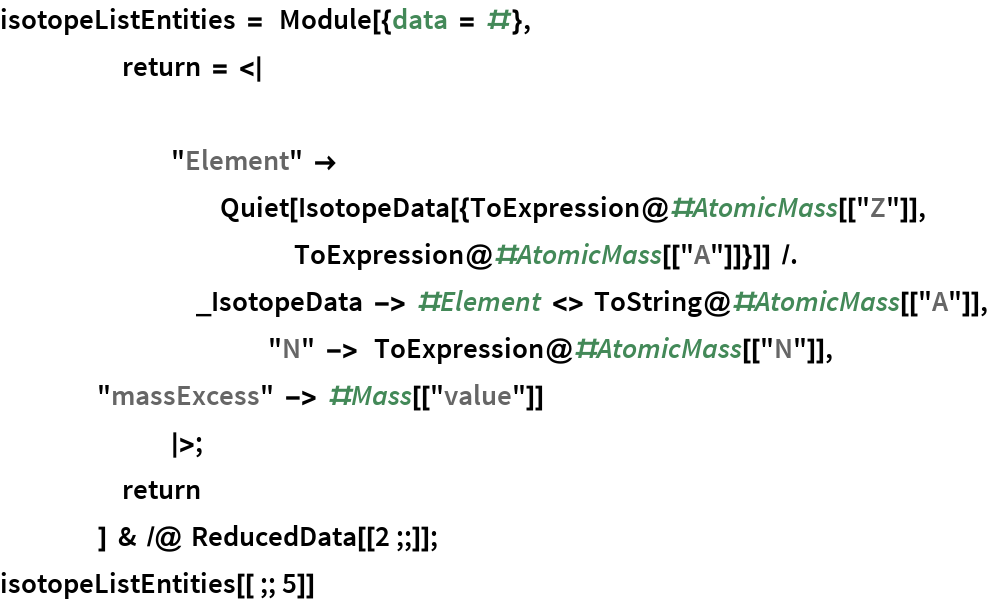Out=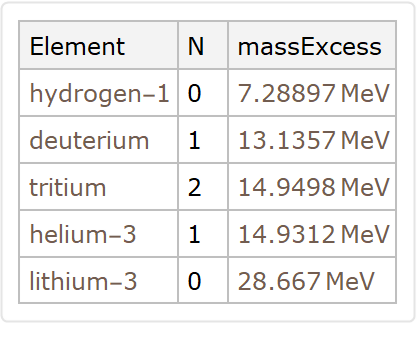The element that aren't entities in Wolfram Language were set with a name as a string. Let`s query them:

 In:=Out=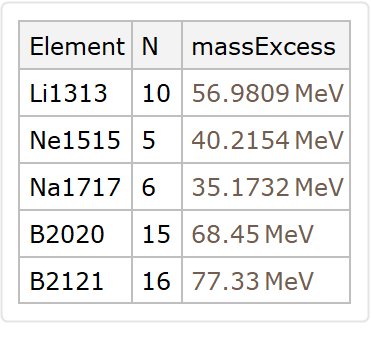How many elements are missing on WL? Let`s check:

 In:=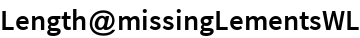Out=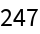We have the list with all elements and the missing elements. We plot it differentiating the missing ones as the red dots.

 In:=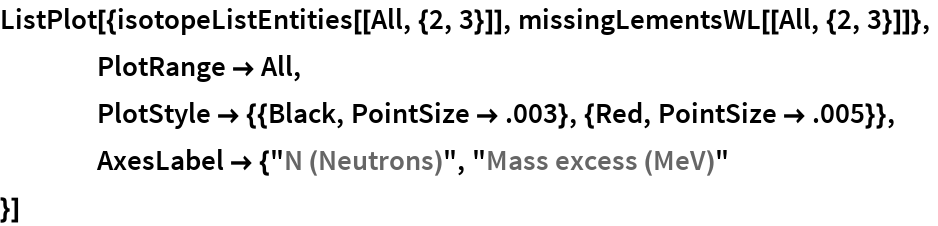Out=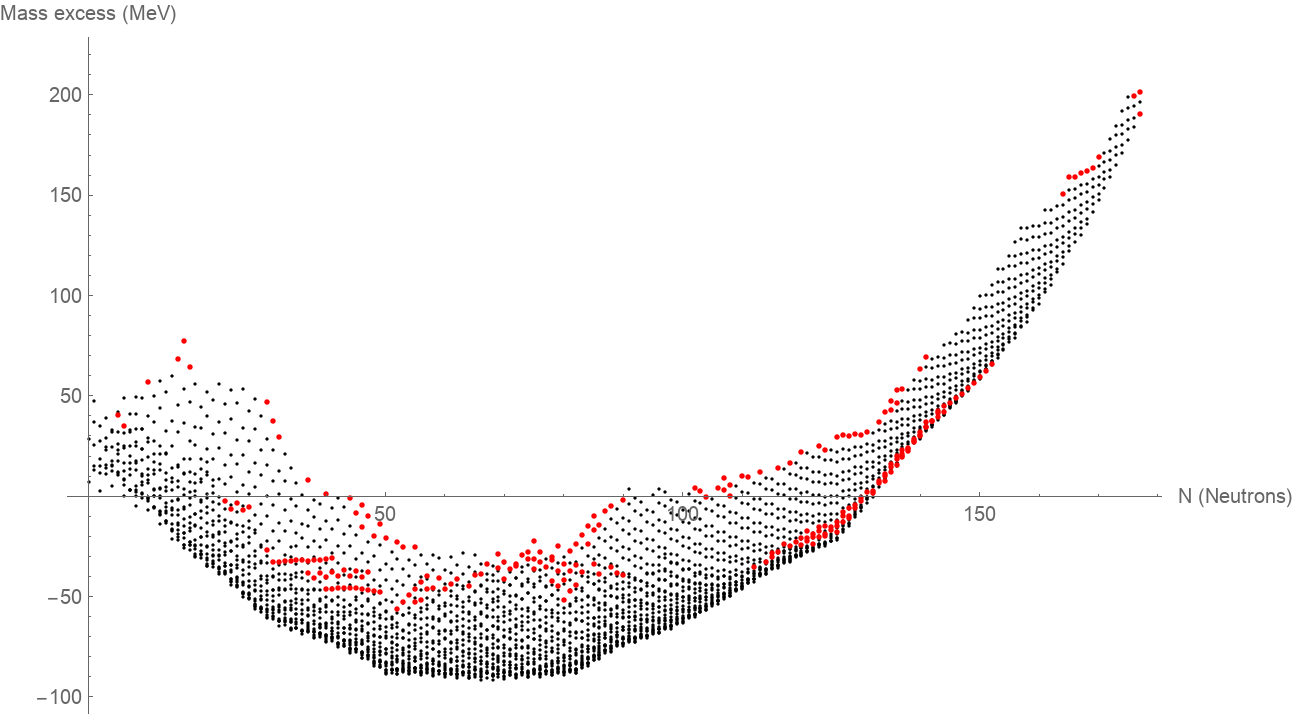### Analysis

One can conclude that WL misses 247 elements if we compare the latest data with the one available on the knowledge base. This data resource will be of good use for atomic, nuclear physics and related areas.

Estevao A Teixeira, "The 2016 Atomic Mass Evaluation (AME2016)" from the Wolfram Data Repository (2020)